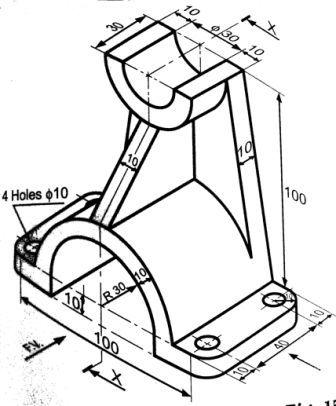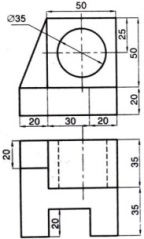MORE IN Engineering Graphics
GTU First Year Engineering (Semester 1)
Engineering Graphics
December 2013
Total marks: --
Total time: --
INSTRUCTIONS
(1) Assume appropriate data and state your reasons
(2) Marks are given to the right of every question
(3) Draw neat diagrams wherever necessary

Choose appropriate answer from the given options for the following:
1 (a) (i) In the first angle projection method, the view seen from left is placed on
(a) Above Front View
(b) Right of Front View
(c) Above Top View
(d) Below Front View
1 M
1 (a) (ii) While drawing the isometric view of the sphere, its diameter is taken as
(a) Equal to actual diameter
(b) 11/9 times of the actual diameter
(c) 21/9 times of the actual diameter
(d) none of the above
1 M
1 (a) (iii) A French curve is used to draw
(a) Circles
(b) Ellipses
(c) Smooth freefrom curves
(d) Polygon
1 M
1 (a) (iv) Which one of the following is not a reduction scale?
(a) 1:1
(b) 1:200
(c) 5/320
(d) 5:6
1 M
1 (a) (v) If the generating point is on the generating circle and the generating circle is outside the directing circle, the curve obtained is:
(a) Inferior hypotrochoid
(b) epicycloids
(c) hypocycloid
(d) superior trochoid
1 M
1 (a) (vi) To obtain the true shape of the section of solid, an auxiliary plane is set
(a) Inclined at an angle of 45° to a cutting plane
(b) parallel to XY
(c) Parallel to a cutting plane
(d) perpendicular to a cutting plane
1 M
1 (a) (vii) If a line is inclined to the Vertical Plane and parallel to Horizontal Plane, then which of the following statements is always CORRECT?
(a) True Length = Plan Length
(b) True Length ? Plan Length
(c) True Length > Elevation Length
(d) True Length = Elevation Length
1 M
State true or false for the following sentences.
1 (b) (i) Dashed lines are used to showoutlines of the adjacent part.
1 M
1 (b) (ii) Measurement of the distances is simpler ina diagonal scale thana vernier scale.
1 M
1 (b) (iii) If front view of a line is parallel toXY, its Top View gives True Length
1 M
1 (b) (iv) The eccentricity of parabola is less than the eccentricity of a hyperbola.
1 M
1 (b) (v) A horizontal section plane will create a sectional Front View
1 M
1 (b) (vi) If a point is in the Vertical Plane, its Front View is seen on XY
1 M
1 (b) (vii) A tetrahedron has four equal square faces.
1 M

2 (a) Construct the parabola if the base is 105 mm and the axis length is 98 mm using rectangle method. Locate focus, vertex and directrix of the parabola.
7 M
2 (b) A wheel of diameter 60 cm, rolls on a straight horizontal road. Draw the locus of a point P on the periphery of the wheel for one revolution of the wheel, if P is initially on the road. Name the curve.
7 M

3 (a) A line AB, 100 mm long, is inclined at 50°to Horizontal Plane. The end A is 10 mm above the Horizontal Plane and end is 65 mm in front of the Vertical Plane. Draw projections of the line if its Front View measures 90 mm and find the inclination of the line with the Vertical Plane.
7 M
3 (b) A line AB is 80 mm ling. It is inclined at an angle of 45°to the Horizontal Plane and 30° to the Vertical Plane. The end A is 20 mm above Horizontal Plane and infront of Vertical Plane. Draw the projections of the line and alsowrite Elevation Length and Plan length of the line.
7 M

4 (a) A semi-circular thin plate of 60 mm diameter, rests on the Horizontal Plane on its diameter, which is inclined at 45°to the Vertical Plane and the surface is inclined at 30°to the Horizontal plane. Draw the projections of the plate.
7 M
4 (b) A pentagonal pyramid of 35 mm base edge and 70 mm height is resting on the Horizontal Plane with one of its triangular surfaces perpendicular to the Horizontal Plane and parallel and nearer to Vertical Plane. Draw its projections.
7 M

5 (a) A tetrahedron of 70 mm long edges is lying on Horizontal Plane on one of its faces with an edge of that face perpendicular to the Vertical Plane. It is cut by a section plane perpendicular to both the reference plane in sucha way that the true shape of section is an isosceles triangle of 45 mm height. Draw levation, plan and side view when smaller cut piece of the object is assumed to be removed
7 M
5 (b) A rhombus is having its diagonals 100 mm and 50 mm long. Drawthe projections of the rhombus when the longer diagonal is inclined at 30°to the Horizontal Plane and 30°to Vertical Plane.
7 M

6 (a) In orthographic projection why second and fourth angle projection method are not used ?
2 M
6 (b) Pictorial view of an object is given in figure 1. Draw(i) Front View and (ii) Full Sectional Right Hand Side View. Insert necessary dimensions using aligned system of dimensioning. Take section along X-X12 M

7 (a) Differentiate between Isometric View and Isometric Projections.
2 M
7 (b) Draw the isometric view from the orthographic projections shown in figure 212 M

More question papers from Engineering Graphics